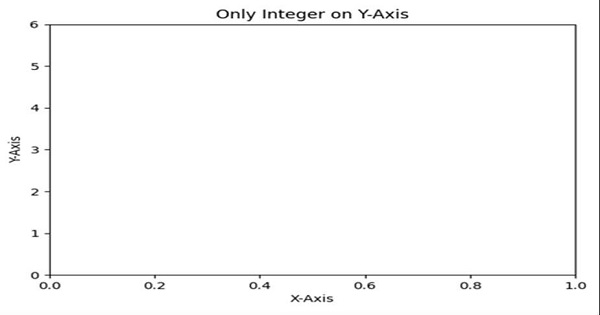# How to force the Y axis to use only integers in Matplotlib?

Whenever Y value list will be made, then we will convert those datasets into a new list, with ceil and floor value of the given list accordingly. Then, we can plot the graph for the new list data.

## Steps

• Take an input list.

• Find the minimum and maximum values in the input list (Step 1).

• Create a range between min and max value (Step 2).

• Get or set the current tick locations and labels of the Y-axis, with a new list.

• Set the X-axis label using plt.xlabel() method.

• Set the Y-axis label using plt.ylabel() method.

• Set a title for the axes.

• To show the figure we can use the plt.show() method.

## Example

import math
from matplotlib import pyplot as plt

y = [0.17, 1.17, 2.98, 3.15, 4.11, 5.151]

minimum_ele = min(y)
maximum_ele = max(y)
new_list = range(math.floor(min(y)), math.ceil(max(y))+1)

plt.yticks(new_list)
plt.xlabel("X-axis ")
plt.ylabel("Y-axis ")
plt.title("Only Integer on Y-axis ")
plt.show()

## Output